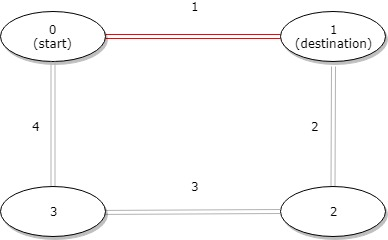LeetCode - Arrays - Distance between Bus Stops

A bus has n stops numbered from 0 to n - 1 that form a circle. We know the distance between all pairs of neighboring stops where distance[i] is the distance between the stops number i and (i + 1) % n.

The bus goes along both directions i.e. clockwise and counterclockwise.

Return the shortest distance between the given start and destination stops.

Example 1:Input: distance = [1,2,3,4], start = 0, destination = 1
Output: 1
Explanation: Distance between 0 and 1 is 1 or 9, minimum is 1.

class Solution {
public int distanceBetweenBusStops(int[] distance, int start, int destination) {
int cw_dist = 0;
int acw_dist = 0;
if(start > destination) {
int temp = start;
start = destination;
destination = temp;
}
for(int i=start;i<destination;i++){
cw_dist = cw_dist+distance[i];
}
//System.out.println(cw_dist);

int j=0;
int counter = destination;
while(j<distance.length){
if(counter > distance.length-1){
counter = 0;
}
if(counter == start || cw_dist<=acw_dist) break;
acw_dist = acw_dist + distance[counter];
counter++;
j++;
}
//System.out.println(acw_dist);

return (cw_dist<=acw_dist)?cw_dist:acw_dist;
}
}